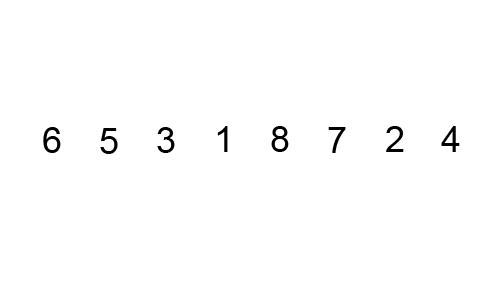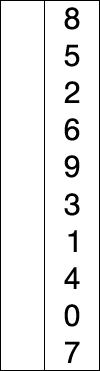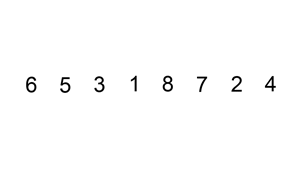# Ricardo Borges

Personal blog## Starting with sorting algorithms

a sorting algorithm is an algorithm that sorts elements in a list into an order, among other uses, sorting can be applied to prepare a set of data for another algorithm, for example, Binary Search.

In this post, I'll describe three sorting algorithms that, although not the most efficient, are easy to understand and are in-place algorithms, meaning that they don't require auxiliary data structures.

### Bubble Sort

Bubble sort starts at the beginning of the list, comparing each pair of adjacent elements, if the first is greater than the second, it swaps them. Then it starts again at the beginning of the list and repeats this process until no swap occurred on the last pass.``````1function swap(arr: number[], i: number, j: number) {
2  [arr[i], arr[j]] = [arr[j], arr[i]];
3}
4
5function bubbleSort(arr: number[]) {
6  for (let i = 0; i < arr.length - 1; i++) {
7    let swapped = false;
8
9    for (let j = 0; j < arr.length - 1; j++) {
10      if (arr[j] > arr[j + 1]) {
11        swap(arr, j, j + 1);
12        swapped = true;
13      }
14    }
15
16    if (!swapped) break;
17  }
18}
19
20const arr = [5, 3, 1, 2, 4];
21bubbleSort(arr);
22console.log(arr);``````

Since its time complexity is Ο(n^2) on average and worst cases, this algorithm is more suitable for small or nearly ordered data sets.

### Selection Sort

The list is divided into a sorted part at the left and an unsorted part at the right in this algorithm. Initially, the sorted sublist is empty and the unsorted one is all the list. Then it searches for the smallest element in the unsorted sublist and swaps it with the leftmost unsorted element, moving the sublist boundaries one element to the right.``````1function selectionSort(arr: number[]) {
2  for (let i = 0; i < arr.length - 1; i++) {
3    let min = i;
4
5    for (let j = i + 1; j < arr.length; j++) {
6      if (arr[j] < arr[min]) {
7        min = j;
8      }
9    }
10
11    swap(arr, min, i);
12  }
13}
``````

Like Bubble Sort, this algorithm has a quadratic time complexity Ο(n^2), so it is also more suitable for small or nearly ordered data sets. However, Selection Sort performs fewer swaps than Bubble Sort.

### Insertion Sort

Insertion Sort keeps a sorted sublist at the beginning of the list, and for each element from the list, it searches for the right position in that sublist to insert that element.``````1function insertionSort(arr: number[]) {
2  for (let i = 1; i < arr.length; i++) {
3    let value = arr[i];
4    let j = i - 1;
5
6    while (j >= 0 && arr[j] > value) {
7      arr[j + 1] = arr[j];
8      j = j - 1;
9    }
10    arr[j + 1] = value;
11  }
12}
``````

Insertion sort has a quadratic time complexity for average and worst cases too, however, it's the fastest sorting algorithm for small lists.